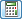Join one of our upcoming courses taking place around the world! Explore now

# Constant Field Stress

Use the Constant field stress option to define a constant stress field prior to excavation (i.e. one which does not vary with position or depth). Constant field stress is typically used for excavations which are relatively deep (i.e. not close to the ground surface).

In the Field Stress dialog, the following parameters are used to define a Constant field stress:

• Sigma 1 is the MAJOR IN-PLANE principal field stress. See note below about the Stress Transformtool.
• Sigma 3 is the MINOR IN-PLANE principal field stress.
• Sigma Z is the OUT-OF-PLANE field stress (i.e. the axis pointing into the screen).

## Angle

The Angle is measured between the positive x-axis and the direction of Sigma 1. The Angle is measured in degrees, and the counter-clockwise direction is defined as positive.

Note: remember that the Stress Block indicates the relative magnitude and direction of the In-Plane principal field stresses. If the Stress Block looks wrong then try entering the field stress data again, keeping in mind the definition of Angle. The display of the Stress Block can be toggled on or off in the View menu.

## Custom Field Stress

Customized field stress may be defined for individual materials in a model, by selecting the Advanced button and defining custom field stress as required. See the Custom Field Stress topic for details.

## Stress Transformation Tool

If your measured 3-dimensional principal stress field is not aligned with the axes of your 2-dimensional plane strain RS2 model, you can use the Stress Transformoption, located beside the Sigma 1 input edit box in the Field Stress dialog, to convert the 3D stress field into an equivalent plane strain principal stress field aligned with the axes of your model.

Select the Stress Transformcalculator button, and you will see another dialog. Enter the following:

1. Tunnel Orientation - enter the 3-dimensional Trend / Plunge of the tunnel or excavation axis (i.e. the Trend / Plunge of the Z-axis of the 2-dimensional plane strain model, which points into the screen).
2. Stress Orientation - enter the 3-dimensional principal stress magnitudes and the Trend / Plunge of the major and minor 3D principal stresses Sigma 1 and Sigma 3. The Sigma 2 axis is automatically assumed to be orthogonal to the Sigma 1 / Sigma 3 plane.
3. Note: the Trend / Plunge of Sigma 1 and Sigma 3 must be orthogonal to each other, otherwise you will see a popup warning message when you select Compute.

4. Compute - select the Compute button in the Stress Transform dialog. This will automatically transform the 3D principal stress field, into a plane strain stress field aligned with the axes of your excavation.
5. Plane Strain Principal Stresses - after selecting Compute, the plane strain principal stresses (Sigma 1 and Sigma 3 in-plane and Sigma Z out-of-plane) and angle of Sigma 1 (in the XY plane) are calculated.
6. Select OK in the Stress Transform dialog, and the calculated plane strain stress field will be automatically loaded into the Field Stress dialog.

Note: the Stress Transform tool cannot be used with Axisymmetric analysis, it is only applicable for Plane Strain analysis.

## Axisymmetric Constant Field Stress

If you are modeling an Axisymmetric problem (this is set in the Project Settings dialog), then the Field Stress dialog will ask you for:

• Horizontal Stress
• Vertical Stress

(rather than Sigma 1, Sigma 3, Sigma Z and Angle).

It is important to note that Vertical and Horizontal refer to the setup of your problem in RS2, and not necessarily the true orientation of your excavation. The Vertical stress is the stress in the axial direction (i.e. the axis of rotational symmetry), and the Horizontal stress is the field stress perpendicular to the axis of symmetry. The terms Vertical and Horizontal are used in RS2 because an axisymmetric model must always be defined such that the axial direction is oriented with the y-axis of the coordinate system.

Out-of-plane (or circumferential) field stress exists, but is automatically set equal to the Horizontal (or radial) stress, and cannot be independently varied by the user.

See the RS2 Tutorials for a tutorial on Axisymmetric modeling.